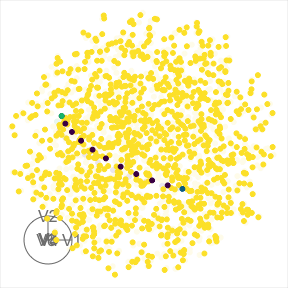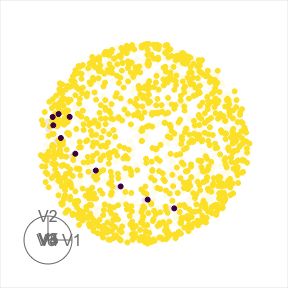# Plotting with woylier

This vignette will demonstrate how to plot the interpolated path with the woylier package.

library(woylier)
library(geozoo)
library(ggplot2)
library(dplyr)
#>
#> Attaching package: 'dplyr'
#> The following objects are masked from 'package:stats':
#>
#>     filter, lag
#> The following objects are masked from 'package:base':
#>
#>     intersect, setdiff, setequal, union
library(purrr)

# 1D example

1D projection of data in high dimension linear combination of data that is normalized. Therefore, we can plot the point on the surface of a hypersphere. The plot below the Givens interpolation steps between 2 points, 1D projection of 6D data that is.

# Generate 1D example
set.seed(2022)
p <- 6
base1 <- tourr::basis_random(p, d=1)
base2 <- tourr::basis_random(p, d=1)

# First example
frames <- givens_full_path(base1, base2, nsteps = 10)
sp <- generate_space_view(p=p)
point1$type <- "point1" point2 <- as.data.frame(t(base2)) point2$type <- "point2"
tourr::animate_xy(sp_path[,1:p], col=sp_path$type, axes="bottomleft") #> Converting input data to the required matrix format. #> Using half_range 0.55# 2D example In case of 2D projections, we can plot the interpolated path between 2 frames on the surface of torus. Torus can be seen as crossing of 2 circles that are orthonormal. # Generate 2D example set.seed(2022) n <- 1000 p <- 3 d <- 2 base1 <- tourr::basis_random(p, d) base2 <- tourr::basis_random(p, d) frames_2d <- givens_full_path(base1, base2, 10) proj_2d <- map(1:n, ~tourr::basis_random(n = p, d=d)) %>% purrr::flatten_dbl() %>% matrix(ncol = p*2, byrow = TRUE) %>% as_tibble() #> Warning: The x argument of as_tibble.matrix() must have unique column names if #> .name_repair is omitted as of tibble 2.0.0. #> ℹ Using compatibility .name_repair. # Path path_2d <- t(apply(frames_2d, 3, c)) %>% as.data.frame() # Join proj_2d <- proj_2d %>% mutate(type="torus") path_2d <- path_2d %>% mutate(type="path") proj_path <- bind_rows(proj_2d, path_2d) tourr::animate_xy(proj_path[,1:6], col=proj_path$type,
#> Using half_range 0.73